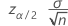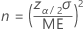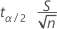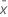# Methods and formulas for Sample Size for Estimation

Select the method or formula of your choice.

## Mean (Normal)

### Standard deviation is known

The confidence interval for a mean from a normal distribution when the population standard deviation is known is:

The margin of error isTo solve for n:### Standard deviation is not known

The confidence interval for a mean from a normal distribution when the population standard deviation is unknown is:

The margin of error isTo solve for n, calculate the minimum n such that:

### Notation

TermDescriptionsample mean
zα/2 inverse cumulative probability of the standard normal distribution at 1- α /2; α = 1 - confidence level/100
σ population standard deviation (assumed known)
n sample size
MEmargin of error
t α/2 inverse cumulative probability of a t distribution with n-1 degrees of freedom at 1-α/2
S planning value

## Proportion (Binomial)

### Upper limit

The interval (PL, PU) is an approximate 100(1 – α)% confidence interval of p.

###### Note

When x = 0 or x = n, Minitab calculates only a one-sided confidence interval.

The lower margin of error equals −1 × (lower bound confidence limit). The upper margin of error equals the upper bound confidence limit.
To solve for n, calculate the minimum n such that:
(P – PL) ≤ ME and (PU – P) ≤ ME where P = planning value proportion.

### Notation

TermDescription
v1 (lower limit) 2x
v2 (lower limit) 2(nx + 1)
v1 (upper limit)2(x + 1)
v2 (upper limit)2(nx)
x number of events
n number of trials
F upper α/2 point of F distribution with v1 and v2 degrees of freedom

## Rate and Mean (Poisson)

### Formula

The lower bound confidence limit for a rate or mean from a Poisson distribution is:

The upper bound confidence limit for a rate or mean from a Poisson distribution is:

The lower margin of error equals −1 × (lower bound confidence limit). The upper margin of error equals the upper bound confidence limit.

To solve for n, calculate the minimum n such that:

(SSL) ≤ ME and (SUS) ≤ ME

### Notation

TermDescription
n sample size
t length of observation; for the Poisson mean, length = 1
s total number of occurrences in a Poisson process
χ2p, x upper x percentile point of a chi-square distribution with p degrees of freedom, where 0 < x < 1
S planning value
MEmargin of error

## Variance and Standard Deviation (Normal)

### Formula

The lower bound confidence limit for variance from a normal distribution is:
The upper bound confidence limit for variance from a normal distribution is:

To obtain the confidence interval for the standard deviation, take the square root of the above equations.

The lower margin of error equals −1 × (lower bound confidence limit). The upper margin of error equals the upper bound confidence limit.

To solve for n for variance, calculate the minimum n such that:

(S2S2L) ≤ ME and (S2US2) ≤ ME

To solve for n for standard deviation, calculate the minimum n such that:

(SSL) ≤ ME and (SUS) ≤ ME

### Notation

TermDescription
n sample size
s2 sample variance
Χ2 p upper 100pth percentile point on a chi-square distribution with (n – 1) degrees of freedom
S planning value
MEmargin of error
By using this site you agree to the use of cookies for analytics and personalized content.  Read our policy